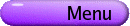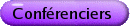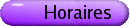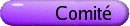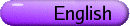Combinatoire
Org: Peter Dukes et Frank Ruskey (Victoria)
[PDF]

KAROLY BEZDEK, University of Calgary, 2500 University Drive N.W., Calgary, AB T2N 1N4
The illumination problem and the cube
[PDF]

Let K be an arbitrary convex body in d-dimensional Euclidean space and let -1 < k < d be some fixed nonnegative integer. Then let I(k,K) denote the smallest number of k-dimensional affine subspaces that illuminate K. According to a conjecture of K. Bezdek (1994), if C denotes the d-dimensional unit cube, then I(k,K) is always at most as large as I(k,C). In the talk we survey the status of this conjecture including the more recent results on the rather combinatorial quantity I(k,C).

ILIYA BLUSKOV, University of Northern BC
Pair Covering Designs with Block Size 6
[PDF]

A t-(v,k,l) covering design, denoted (V,B), where v=|V|, is a finite family B of k-subsets of V, called blocks, such that each t-subset of V occurs in at least l blocks. The covering number Cl(v,k,t) is min|B|, where the minimum is taken over all t-(v,k,l) covering designs. My talk is based on a recent joint work (with Abel, Greig and de Heer) on the covering number C1(v,6,2). This number meets the Schönheim bound:

 C1(v,k,2) ³ éê v k éê v-1 k-1 ùú ùú .
We show that C1(v,6,2) attains the Schönheim bound for all v º 2 mod 5. I will discuss direct combinatorial constructions and computer assisted searches related to this result.

AIDEN BRUEN, U. of Calgary, Dept. of Mathematics and Statistics
Combinatorial characterizations of perfect secrecy
[PDF]

We discuss entropy in classical information theory and Shannon's concept of perfect secrecy in cryptography. Examples include the Vernam cipher, also known as the one-time pad. Under suitable conditions we prove the equivalence of perfect secrecy with a well-known class of combinatorial structures. We then proceed to discuss analagous questions in quantum information theory. This in turn leads to a much-studied and fundamental classical question in combinatorics dating back to Euler. We conclude, time permitting, with some "philosophical musings".

JONATHAN JEDWAB, Simon Fraser University, 8888 University Drive, Burnaby, BC V5A 1S6
Proof of the Barker array conjecture
[PDF]

Using only elementary methods, we prove Alquaddoomi and Scholtz's conjecture of 1989, that no s ×t Barker array having s, t > 1 exists except when s = t = 2.

Joint work with J. A. Davis and K. W. Smith.

VESELIN JUNGIC, Simon Fraser University, Burnaby, BC
Ramsey Rainbow Theory
[PDF]

The van der Waerden theorem in Ramsey theory states that, for every k and t and sufficiently large N, every k-coloring of [N] contains a monochromatic arithmetic progression of length t. Motivated by this result, Radoici\'c conjectured in 2001 that every equinumerous 3-coloring of [3n] contains a 3-term rainbow arithmetic progression, i.e., an arithmetic progression whose terms are colored with distinct colors. This conjecture initiated a serious results having rainbow structures as the common theme. One such result is that every 3-coloring of the set of natural numbers for which each color class has density more than 1/6, contains a 3-term rainbow arithmetic progression. A similar results for colorings of Zn is true.

In this presentation an overview of the current state in research directions in the rainbow Ramsey theory will be given. I will list results, problems, and conjectures related to existence of rainbow arithmetic progressions in [n] and N. A general perspective on other rainbow Ramsey-type problems will be given.

MARK KAYLL, University of Montana, Missoula, MT, USA
A variation of chip firing
[PDF]

A chip-firing game on a graph G=(V,E) begins by placing a configuration C: V®N of chips on its vertices. Then single chips are added sequentially to vertices selected uniformly at random. If, after any step, a vertex v contains more chips than its threshold, i.e., if C(v) > deg(v), then v fires, sending one chip to each neighbor and losing one chip to the `ether'. A firing event may trigger subsequent firings; these play out as long as possible-until the chip configuration is `relaxed'-at which point a new vertex is seeded, and the process repeats. This talk surveys some results on this version of chip firing, a variant on one studied by Bjorner, Lovász, Shor and others in the early 1990s. A sample (perhaps surprising) result: if the relaxed configurations are viewed as states in a Markov chain, then its stationary distribution is uniform over all `legal' configurations.

This joint work with David Perkins (Houghton College, NY) forms the basis for his recent PhD thesis.

[PDF]

A regular Hadamard matrix with row sum 2h is called productive if there is a set H of matrices with row sum 2h and a cyclic group G = \precs\succ where s: H ® H is a bijection, such that

1. H Î H,

2. For any H1,H2 Î H, (sH1)(sH2)t = H1 H2t,

3. |G|=4|h|,

4. åq Î G qH = 2[(h)/(|h|)] J.

We show that for each integer n for which there is a Hadamard matrix of order 4n and 8n2-1 is a prime number, there is a productive regular Hadamard matrix of order 16n2(8n2-1)2. Applications include a new class of symmetric designs.

This is a joint work with Majid Behbahani.

ESTHER LAMKEN, c/o Dept. of Math, UC Berkeley, Berkeley, CA
A few more Kirkman squares with block size 3
[PDF]

In a series of papers, I established the existence of Kirkman squares with block size 3 for (m,l) = (1,2), (2,4) and the existence of doubly near resolvable (v,3,2)-BIBDs with a small number of exceptions. Recently, Julian Abel, Jinhua Wang, and I have constructed designs for all of the open cases. In addition, we constructed several more Kirkman squares with block size 3 and m = l = 1. In this talk, I will describe some of our new constructions.

PIERRE LEROUX, Université du Québec à Montréal, LaCIM et Département de mathématiques
Characterization and enumeration of projective-planar and toroidal K3,3-subdivision-free graphs
[PDF]

In his 2003 Ph.D thesis at University of Manitoba, Andrei Gagarin has studied graph embeddability on the projective plane and the torus, from an algorithmic point of view, particularly when avoiding K3,3-subdivision. Building on his results, we have been able to determine completely the structure of projective planar and toroidal K3,3-subdivision-free graphs and to enumerate them.

Their characterization is expressed in terms of substitution of 2-pole planar networks for the edges of canonically defined non-planar graphs called projective-planar cores and toroidal cores respectively.

Their enumeration (both labelled and unlabelled) is achieved by using methods developed by T. Walsh in 1982 for edge substitutions in the context of 3-connected and homeomorphically irreducible 2-connected graphs.

This is joint work with Andrei Gagarin and Gilbert Labelle.

PETR LISONEK, Department of Mathematics, Simon Fraser University, Burnaby, BC V5A 1S6
Combinatorial families enumerated by quasi-polynomials
[PDF]

We say that the sequence (an) is quasi-polynomial in n if there exist polynomials P0,...,Ps-1 such that an=Pi(n) where i º n mod s. We present several families of combinatorial structures with the following properties: Each family of structures depends on two or more parameters, and the number of isomorphism types of structures is quasi-polynomial in one of the parameters whenever the values of the remaining parameters are fixed to arbitrary constants. For each family we are able to translate the problem of counting isomorphism types of structures to the problem of counting integer points in a union of parameterized rational polytopes. The quasi-polynomiality of the counting sequence then follows from Ehrhart's result about the number of integer points in the sequence of integral dilates of a given rational polytope. The families of structures to which this approach is applicable include combinatorial designs, linear and unrestricted codes, and dissections of regular polygons.

ERIC MENDELSOHN, University of Toronto
When Systems Collide
[PDF]

We present a conjecture which is a common generalization of the Doyen-Wilson Theorem and Lindner and Rosa's intersection theorem for Steiner triple systems. Given u,v, º 1,3 mod 6, u < v < 2u+1 we ask for the minimum r such that there exists a Steiner triple system (U,B), |U|=u such that some partial system (U,B \\pmb\mathfrak d) can be completed to a STS(v), (V,B¢), where |\pmb\mathfrak d| = r. In other words, in order to "quasi-embed" an STS(u) into an STS(v), we must remove r blocks from the small system, and this r is the least such with this property. One can also view the quantity u(u-1)/6-r as the maximum intersection of an STS(u) and an STS(v) with u < v. We conjecture that the necessary minimum r = (v-u)(2u+1-v)/6 can be achieved, except when u=6t+1 and v=6t+3, in which case it is r = 3t for t ¹ 2, or r=7 when t=2. Using small examples and recursion, we solve the cases v-u=2 and 4, asymptotically solve the cases v-u=6,8,10, and further show for given v-u > 2 that an asymptotic solution exists if solutions exist for a run of consecutive values of u (whose required length is no more than v-u). Some results are obtained for v close to 2u+1 as well. The cases where v » 3u/2 seem to be the hardest. For intersections sizes between 0 and this maximum we generalize Lindner and Rosa's intersection problem-"determine the possible numbers of blocks common to two Steiner triple systems STS(u), (U,B), U=V" to the cases STS(v), (V,B¢), with U Í V and solve it completely for v-u=2,4 and for v ³ 2u-3.

Joint work with P. Dukes.

MARNI MISHNA, Simon Fraser University, 8888 University Drive, Burnaby, BC
Walks in the quarter plane
[PDF]

We consider two-dimensional lattice walks, with a fixed set of step directions, restricted to the first quadrant. These walks are well studied, both in a general context of probabilistic models, and specifically as particular case studies for particular cases of direction sets. The goal here is to examine two series associated to these walks: a simple length generating function, and a complete generating function which encodes endpoints of walks, and to determine combinatorial criteria which decide when these series are algebraic, D-finite, or none of the above. We shall present an (almost) complete classification of all nearest neighbour walks where the set of directions is of cardinality three, and discuss how this leads to a natural, well supported, conjecture for the classification of nearest walks with any direction set.

Work in progress with M. Bousquet-Melou.

DANIEL PANARIO, School of Mathematics and Statistics, Carleton University, 1125 Colonel By Drive, Ottawa, ON K1S 5B6
Asymptotics of largest and smallest components in combinatorial structures
[PDF]

We study the extremal size of components in decomposable combinatorial structures. We have in mind combinatorial objects such as permutations that decompose into cycles, graphs into connected components, polynomials over finite fields into irreducible factors and so on. The probability that the size of the smallest components of combinatorial objects of size n be at least m is explained by the Buchstab function, as shown by Panario and Richmond (2001) using the Flajolet-Odlyzko singularity analysis method. In this talk, we adapt Buchstab's recursive arguments for integers to combinatorial objects. We study the probability of connectedness for structures of size n when all components have size at least m. In the border between almost certain connectedness and almost certain disconnectedness, we encounter a generalized Buchstab function.

For largest components, using singularity analysis, Gourdon (1996) has studied the probability that structures of size n have all components of size at most m. We also give a recursive argument for the probability of connectedness for structures of size n when all components have size at most m. In this case, our results are given in terms of a generalized Dickman function. The Dickman function appears when studying the largest prime factor of a random integer between 1 and n.

We apply these results to several combinatorial structures such as permutations, polynomials over finite fields, labelled 2-regular graphs, functional digraphs, trees (unrooted and rooted case), labelled and unlabelled graphs, Achiral trees, and k-point labelled stars.

Based on joint works with: Ed Bender, Atefeh Mashatan and Bruce Richmond (JCTA 2004) and Mohamed Omar, Bruce Richmond and Jacki Whitely (to appear in Algorithmica).

ANDREW RECHNITZER, University of Melbourne
Disproving things is easier when you know they are false-experimenting with two conjectures on pattern-avoiding permutations
[PDF]

Perhaps the most important mathematical idea that I have learnt was that "It is always easier to prove something when you know it is true."

I have used this idea a great deal in my work; computer-aided number crunching has been a crucial part of many of my results.

Recently I was introduced the problem of counting pattern-avoiding permutations, which arises in different contexts in computer science and algebra. In the last decade or so it has been subject to a great deal of work and a number of conjectures have been made. Some of these have recently been proved, but much work remains to be done.

In this talk I will give a quick discussion of pattern-avoiding permutations before focussing on some of the experimental work I have done which led me to realise (but not prove) that two open conjectures were false. Thankfully it is easier to disprove something when you know it is false, and I will show you how we disproved one conjecture and (given time) some of our work towards disproving the other.

FRANK RUSKEY, University of Victoria, Victoria, BC
Counting strings over the integers mod a power of two with given elementary symmetric function evaluations
[PDF]

Let a be a string over Zq, where q = 2d. The j-th elementary symmetric function evaluated at a is denoted ej(a). We study the cardinalities Sq (m;t1,t2,...,tt) of the set of length m strings for which ei(a) = ti. The profile k(a) = ák1,k2,...,kq-1 ñ of a string a is the sequence of frequencies with which each letter occurs. The profile of a determines ej(a), and hence Sq. Let hn: Z2n+d-1(q-1) ® Z2d[z] mod z2n be the map that takes k(a) mod 2n+d-1 to the polynomial 1 + e1(a) z + e2(a) z2 + ¼+e2n-1(a) z2n-1. We show that hn is a group homomorphism and establish necessary conditions for membership in the kernel for fixed d. The kernel is determined for d = 2,3. The range of hn is described for d = 2. These results are used to "efficiently" compute S4 (m;t1,t2,...,tt).

This is joint research with Bob Miers at UVic.

CARLA SAVAGE, North Carolina State University
Partitions and compositions defined by digraphs
[PDF]

We consider the enumeration of nonnegative integer sequences a1,a2,...,an satisfying a system C inequalities of the form ai ³ aj + bi,j. In this case, C can be modeled by a directed graph with vertices 1,...,n and with an edge from i to j of weight bi,j for each constraint ai ³ aj + bi,j in C. Many familiar systems can be modeled in this way, including ordinary partitions and compositions, plane partitions, monotone triangles, plane partition diamonds, and solid partitions.

We develop special tools tailored to computing the generating function of sequences defined by a digraph and show how to apply these tools strategically to solve some nontrivial enumeration problems in the theory of partitions and compositions. The focus is on deriving a recurrence for the generating function when the digraph has a recursive structure. Part of this process can be (and has been) automated.

This is joint work with Will Davis, Sunyoung Lee, and Erwin D'Souza.

BRETT STEVENS, Carleton University, 1125 Colonel By Dr., Ottawa, ON K1S 5B6
Maximally Pair Separated Round Robin Tournaments: Ordering the Blocks of a Design
[PDF]

In a Round Robin Tournament with multiple edges, we can ask that we schedule the successive games between any given pair as far apart in time as possible. We show that for a cyclic n day, l = 2, tournament schedule on n players it is impossible to ask that successive game for the same pair be at least ën/2 û days apart. However we also show that if we allow a small number to be separated by ë(n-2)/2 û days apart, then such a schedule is possible. These orderings fit into an interesting unifying framework that brings together quite a few previously known results.

STEPHANIE VAN WILLIGENBURG, University of British Columbia
Multiplicity free expansions of Schur P-functions
[PDF]

Schur P functions arise, for example, as the generating function of shifted tableaux, and can be expressed as a linear combination of the better known Schur functions using the shifted Littlewood-Richardson rule.

In this talk we will give specific criteria for when the aforementioned expression is multiplicity free. From here we will apply this result to determine when the multiplicity of an irreducible spin character of the twisted symmetric group in the product of a basic spin character with an irreducible character of the symmetric group is multiplicity free.

This is joint work with Kristin Shaw.